# Multi-Target Learning Algorithm for Solar Radiation Components Forecasting Based on the Desired Tilt Angle of a Solar Energy System

Multi-Target Learning Algorithm for Solar Radiation Components Forecasting Based on the Desired Tilt Angle of a Solar Energy System

Mohammed Ali JallalAbdessalam El Yassini Samira Chabaa Abdelouhab Zeroual Saida Ibnyaich

I2SP Research Team, Physics Department, Faculty of Sciences Semlalia, Cadi Ayyad University, Marrakesh 40000, Morocco

Industrial Engineering Department, National School of Applied Sciences, Ibn Zohr University, Agadir 80000, Morocco

Corresponding Author Email:
mohammedali.jallal@edu.uca.ac.ma
Page:
187-193
|
DOI:
https://doi.org/10.18280/i2m.200402
28 May 2020
|
Accepted:
13 July 2021
|
Published:
31 August 2021
| Citation

OPEN ACCESS

Abstract:

Solar radiation components (SRC) forecasting with different tilt angles plays a key role for planning, managing, and controlling the solar energy system production. To overcome the gaps related to the intermittence and to the absence of SRC data, an accurate predictive model needs to be established. The main goal of the present work is to develop for solar system engineers and grid operators a precise predictive approach based on multi-target learning algorithm to forecast the hourly SRC measurements that is related to the city of Marrakesh (latitude 31°37′N, longitude 08°01′W, elevation 466m), Morocco, received by different inclined solar panels’ surfaces. For this purpose, eight training algorithms (Resilient back Propagation (Rp), One step secant (OSS), Levenberg-Marquardt (LM) Algorithm, Fletcher-Reeves algorithm (Cgf), Polak-Ribiere algorithm (Cgp), Powell-Beale algorithm (Cgb), gradient descent (Gd) algorithm and scaled conjugate gradient algorithm (Scg)) are tested to optimize the developed approach’s parameters. The forecasting results were performed based on the angle of inclination desired by the operator and some accessible meteorological measurements that are recorded at each hour, comprising time variables. The achieved performance demonstrates the stability and the accuracy of the established approach to estimate the hourly SRC time series compared to several recent literature studies.

Keywords:

1. Introduction

Solar energy is a source of abundant energy that depends on the sun. This energy is used to produce electricity from sunlight captured by solar panels. Solar energy is considered to be the most effective, economic, clean, and renewable energy resource. Planning, managing, and controlling of these systems depend on SRC measurements .

During the last years, solar energy plays a vital role in making a rapid transition towards clean energy production, sustainable and intelligent energy supply . Solar radiation components are the key parameters needed to monitor and design solar energy systems . As the absence or the intermittence of SRC data affect directly the control and the management of solar energy systems, many predictive approaches were developed in order to overcome these gaps by performing accurate SRC predictions in the case of solar sensor or acquisition chain damage .

Some efforts have focused on solar radiation time series forecasting for areas where such measurements are not available. Jallal et al. developed a new machine learning based on the hybridization of deep neural networks with the multi-model approach to predict accurately the hourly global solar radiation (GSR) time series for Marrakech, Morocco, using the most pertinent exogenous inputs measured during seven years (2008-2014) . The same authors have developed an effective AI-data driven approach to forecast the global solar radiation measurements at each half-hour based only on endogenous inputs . They have also developed an autoregressive moving average model based on Box- Jenkins approach to enhance the accuracy of daily global solar radiation prediction . Sun et al. developed a new forecasting strategy for solar radiation (SR) time series based on ensemble mode decomposition and clustering algorithm . Garcia-Hinde et al. proposed a learning strategy for SR data regression based on input dimensionality reduction . Bailek et al. predicted diffuse SR measurement over the Algerian Sahara using an empirical approach .

Hence, a precise SRC forecasting technique is needed to plan and control solar energy installations . Among of the applied approaches, artificial intelligence techniques known a large application with promising results in the last two decades , such as the models based on artificial neural network (ANN) , and fuzzy logic methodology . Based on latest pieces of literature, the nonlinear behavior of the SRC time series and the uncertainties associated to weather conditions the creation of an efficient predictive model become a huge challenging problem for engineers and grid operators . Besides, most of the research studies are focused on the application of single output machine learning algorithms to predict just one component of solar radiation time series due to the complexity and divergence of these techniques in multi-target problems during the training process.

The key contributions of this work are:

• A dynamic approach named Elman ANN is applied to approximate the nonlinear behavior and the rapid fluctuations of SRC evolutions;
• Raw meteorological data are used as exogenous inputs to evaluate the efficiency of the developed model;
• The performance of eight optimizers is investigated to select the best training algorithm for our proposal;
• Solar radiation components (global, direct, and diffuse solar radiation) predictions at each tilt angle, which is fixed by the operator was successfully implemented.

The remaining part of this article is organized as follows: The meteorological time series description and Elman artificial neural network are discussed in Section 2. The forecasting results of the multi-targets Elman ANN model are presented and discussed in Section 3. Finally, conclusions are given in Section 4.

2. Materials and Methods

2.1 Meteorological time series description

In the present work, the typical years given by Meteonorm software of the SRC measurements are computed based on the data recorded from 1991 to 2010; and the and air temperature (AT) time series measurements are measured from 2000 to 2009. SRC and AT measurements are recorded in the hourly scale in the city of Marrakesh city, Morocco (Latitude [°] = 31,650, Longitude [°] = -8,017, Altitude [m] = 437) at different angles of inclination (from 0° to 90° with a step of 5°). The typical year of different meteorological parameters are used to train and evaluate the proposed model. The set of the exogenous inputs that is used to build the proposed model was given in Table 1 with their abbreviations and units. The parameters are standardized according to Meteonorm software.

Table 1. Input meteorological parameters

 Exogenous Input variables Abbreviation Unit Month Day of month Day of year Hour of day Air temperature Relative humidity Wind speed Wind direction Precipitation Effective sunshine duration Cloud cover Solar altitude Linke turbidity factor Extraterrestrial solar radiation m dm dy h Ta RH FF DD RR Sd N hs tl $G_{e x}$ - - - - ℃ % m/s ° mm h/day okta ° - W/m2

Table 2 presents the solar radiation components with their abbreviations and units that are used as targets in the present paper.

Table 2. Target solar radiation components

 Target variables Abbreviation Unit Global horizontal solar radiation Direct solar radiation Diffuse solar radiation Gh GBn Gd W/m2 W/m2 W/m2

2.2 Elman artificial neural network (ENN) description

In this paper, the nature-inspired ENN technique is applied for GSR time series forecasting. The ENN model is adopted for its robustness and its dynamic behavior to handle the chaotic patterns of a time series during the training process . Figure 1 shows the design scheme of the ENN model. The model of ENN is usually involves 4 layers (input, hidden, feedback links, and output layers). The feedback links layer is applied to incorporate the past-generated values of the hidden layer’s output as inputs. This technique includes internal delayed feedback connections of order TDL that have a main role to handle the nonlinear patterns into meteorological time series .

With,

n: Number of inputs x.

m: Number of outputs o.

l: Number of hidden neurons and feedback links are,

k: Current iteration.

$w_{1}$: Weight vector that links the input layer to the hidden layer.

$w_{2}$: Weight vector that connect the feedback links layer to the hidden layer.

$w_{3}$: Weight vector that associate the hidden layer to the output layer.

x(k): ENN input vector.

$y_{h}(k)$: Output vector of hidden layer, calculated by (1);

$y_{c}(k)$: Output of the feedback links layer, determined according to (2);

o(k): Output of ENN computed by using (3).

$y_{h}(k)=\Psi\left(w_{2} y_{c}(k)+w_{1} x(k)\right)$    (1)

Or

$y_{c}(k)=y_{h}(k-1)$    (2)

where,

$\Psi$: Hidden layer activation function,

$\Upsilon$: Output layer activation function that is often a linear function.

Several activation functions can be adopted by the ENN model, such as: radial activation function (radbas), logistic sigmoid function (logsig), and hyperbolic function (tansig) .

The Output of the ENN model is calculated as follows:

$o(k)=\Upsilon\left(w_{3} y_{h}(k)\right)$    (3)

ENN adjust its parameters (weights and biases) based on network error minimization represented by (4), using an efficient training optimizer.

$E=\sum_{i=1}^{n}\left(d_{i}-o_{i}\right)^{2}$    (4)

where, $d_{i}$ is the output target.

In the present work, eight training optimizers (Resilient back Propagation (Rp), One step secant (OSS), Levenberg-Marquardt (LM) Algorithm, Fletcher-Reeves algorithm (Cgf), Polak-Ribiere algorithm (Cgp), Powell-Beale algorithm (Cgb), gradient descent (Gd) algorithm and scaled conjugate gradient algorithm (Scg)) are applied to estimate the ENN model parameters [6, 17, 18], with the mean square error (MSE) that is used as a loss function. More details about the training process of artificial neural networks are presented in Ref. .

## 1.png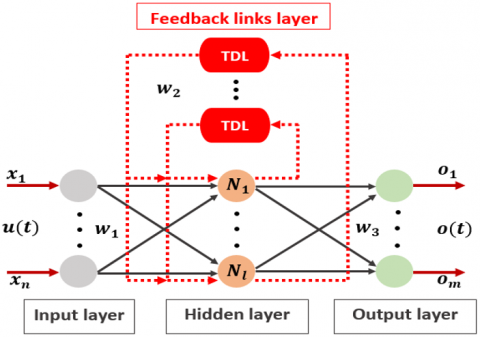Figure 1. Elman neural network design scheme

3. Forecasting Results and Discussions

3.1 Implementation and analysis of the proposed model

In the present study, a multi-targets learning algorithm is proposed to forecast the three components of solar radiations (global, direct, and diffuse) received at any tilt angle desired by the operator. The forecasting results are based on some raw meteorological measurements, including time features as exogenous inputs. These later present their correlation to be applied to forecast the three solar components in different pieces of literature . Subsequently, solar energy systems engineers and grid operators can apply this forecasting approach to perform accurate predictions of the SRC received by a solar energy system’s surface at each tilt angle.

Figure 2 depicts the architecture of the proposed approach. In this study, the performance of training the proposed model using eight optimizers was investigated in order to minimize the difference between the forecasted and the desired targets.

All training algorithms are applied to train the proposed model by using a training dataset of input and output variables, which are associated to the angles of inclinations (0°; 10°; 20°; 30°; 40°; and 50°). The rest of measurements, which are associated to the angles of inclinations (5°; 15°; 25°; 35°; 45°; 55°; 60°; 65°; 70°; 75°; 80°; 85°; and 90°) are used to evaluate the accuracy and the robustness of our proposal.

A trial and error approach is used to determine the ENN model’s hyper-parameters, where there is no deterministic technique to determine them efficiently . For that raison, we have varied the number of hidden layers, number of neurons in the hidden layers and the activation function in order to determine the optimal structure that minimize the mean square error (MSE) metric. More details are presented in .

In Figure 3, the MSE results of the developed model were depicted as a function of hidden layers number. From the attained performance, the single hidden layer is the most suited for the developed predictive model, and the increase of hidden layers number reduce the accuracy of prediction and increase the burden of the computational process.

In Figure 4, the variation of the neurons number in the hidden layer is presented. From the obtained results, the hidden layer with 11 neurons was the best configuration.

By varying the type of activation functions in the hidden layer, the radial basis activation function shows the best performance compared to the other activation function in terms of the MSE indicator.

## 2.pngFigure 2. Basic structure of the developed model

## 3.png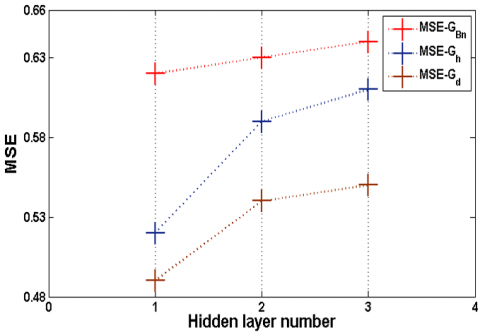Figure 3. Hidden layers number variation as a function of the MSE metric for global, direct, and diffuse solar radiation time series

## 4.png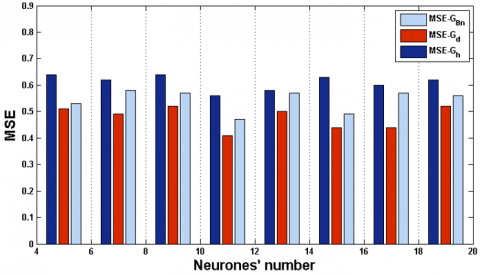Figure 4. Neurons number variation as a function of the MSE metric for global, direct, and diffuse solar radiation time series

The ENN model performance was evaluated using the MSE and the correlation coefficient (R) metrics , which are computed by Eq. (5) and (6).

$M S E=\frac{1}{N} \sum_{i=1}^{N}\left(d_{i}-p_{i}\right)^{2}$    (5)

$R=\sqrt{\sum_{i=1}^{N}\left(p_{i}-d_{m i}\right)^{2} / \sum_{i=1}^{N}\left(d_{i}-d_{m i}\right)^{2}}$    (6)

$p_{i}$: The predicted output;

$d_{i}$: The desired output;

$d_{m i}$: The average value of $d_{i}$;

N: The number of samples.

Subsequently, the influence of the Elman ANN’s feedback links order (TDL) on the forecasting accuracy is investigated by changing its value between 1 and 48. The achieved results in Figure 5, demonstrate that the feedback links’ order 7 is the best value for the current implementation, and its increase distresses the accuracy of the developed model. A small feedback links’ order that ensures a good precision is preferred, when this order increases, the computation complexity of ENN increases too.

After the determination of the predictive model’s configuration, eight backpropagation optimizers are adopted to tune the parameters of the developed model in order to select the best training optimizer for our proposal.

In Table 3, the performance of each training optimizer during the testing phase are presented for each solar radiation components (GBn, Gh, and Gd).

From the R results given by Table 3, the LM optimizer is the most performant one to optimize the developed model parameters. The other training optimizers recorded large differences between the forecasted and the measured SRC time series and less accuracy in terms of the R metric compared to LM optimizer. The attained results allow the application of the LM optimizer to adjust the parameters of the developed model.

In Figure 6, the optimal obtained ENN’s configuration is depicted.

## 5.pngFigure 5. TDL variation as a function of the MSE metric of predicted global, direct, and diffuse solar radiation time series

Table 3. Performance of the training optimizers for forecasting each solar radiation components in terms of the correlation coefficient (R)

 Training optimizer Solar radiation component R(%) Cgp GBn Gh Gd 97.86 97.70 78.49 Cgb GBn Gh Gd 99 99.13 94.16 Cgf GBn Gh Gd 98.81 98.54 90.83 Oss GBn Gh Gd 97.91 97.59 73.85 Gd GBn Gh Gd 1.66 50.04 26.72 Rp GBn Gh Gd 98.80 99.21 96.44 LM GBn Gh Gd 99.8 99.9 99.8

## 6.png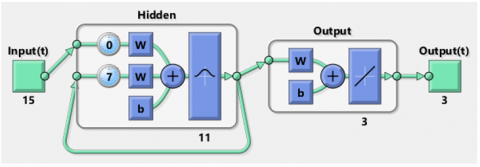Figure 6. Optimal ENN’s structure

3.2 Results and discussion

After determining the optimal Elman ANN model’s configuration. The efficiency in terms of the stability and the accuracy of the developed model need to be evaluated on a new dataset that is not used during the training phase. For this raison, the developed model was applied on other data that are reserved for the testing phase. Figures 7, 8 and 9 depict the scattering graphs of the desired targets (direct, diffuse, and global solar radiation) and their forecasting data with the associated correlation coefficient (R). These assessments provide precise statistics about the correlation existing between the desired and the forecasted time series.

The achieved results demonstrate a high correlation between the predicted and the measured SRC, which prove the efficiency of the proposed approach to forecast the three components of solar radiation, global, direct, and diffuse solar radiation for any tilt angle desired by the operator in one time by using only one model without using a set of three models. Besides, the developed model proves a high accuracy to handle the nonlinear and stochastic comportment of the meteorological time series that are used in this work.

In Table 4, the developed model achieved a high accuracy compared to different forecasting techniques developed in several literature contributions in terms of the correlation coefficient (R).

## 7.pngFigure 7. Scattering diagram of direct solar radiation based on the testing phase

## 8.pngFigure 8. Scattering diagram of diffuse solar radiation based on the testing phase

## 9.png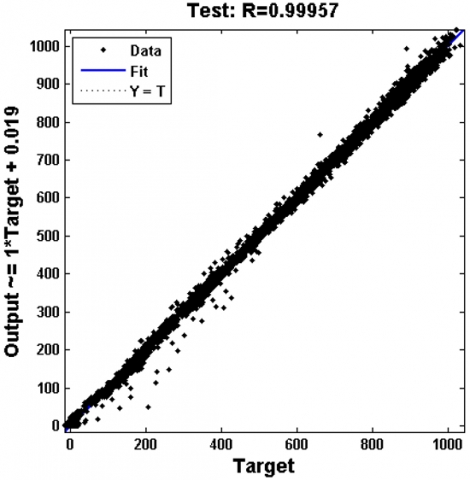Figure 9. Scattering diagram of global solar radiation based on the testing phase

Table 4. Comparison of the proposed approach with well-established forecasting techniques on solar radiation components forecasting

 References locations Parameters Applied methods R (%)  (2019) Beijing, China GSR Empirical model 93  (2019) 90 stations, China GSR ResnetTL 88  (2019) Hong Kong, China GSR MARS 91.3  (2019) Henan, China Diffuse BRL-1 82  (2019) Antakya, Turkey Diffuse DENFIS 97.9  (2020) Wien, Austria Direct Fourier expansions 85.71  (2020) Valentia, Ireland Diffuse Fourier expansions 96.5  (2020) Muğla, Turkey Diffuse HELIOSAT 97.6 Present study (2020) Marrakesh, Morocco Direct Proposed approach 99.8 Present study (2020) Marrakesh, Morocco Diffuse Proposed approach 99.8 Present study (2020) Marrakesh, Morocco GSR Proposed approach 99.9
4. Conclusions

The angle of inclination is an important factor that penalize hardly the energy production of a solar energy system. Therefore, this later need to be adjusted regularly and efficiently to produce the maximum of electricity. In the present paper, a multi-targets learning algorithm is proposed to forecast the three components of solar radiations, global, direct, and diffuse, relative to the city of Marrakesh, Morocco, received by any tilted solar panel’s surface desired by the operator. The forecasting results are based on a set of meteorological parameters and time variables. This kind of forecasting gives a precise information to solar engineers and grid operators about the variation of these solar radiation components by siting the desired angle of inclination. Subsequently, they might predict accurately their targets. The prediction accuracy attained by the developed model demonstrate its efficiency and its robustness to be used by solar energy engineers and electrical grid operators in case of solar sensor or acquisition chain damage.

References

 Mouachi, R., Jallal, M.A., Gharnati, F., Raoufi, M. (2020). Multiobjective sizing of an autonomous hybrid microgrid using a multimodal delayed PSO algorithm: A case study of a fishing village. Comput. Intell. Neurosci., 2020: 8894094. https://doi.org/10.1155/2020/8894094

 Yaniktepe, B., Genc, Y.A. (2015). Establishing new model for predicting the global solar radiation on horizontal surface. Int. J. Hydrogen Energy, 40(44): 15278-15283. https://doi.org/10.1016/j.ijhydene.2015.02.064

 Xue, X. (2017). Prediction of daily diffuse solar radiation using artificial neural networks. Int. J. Hydrogen Energy, 42(47): 28214-28221. https://doi.org/10.1016/j.ijhydene.2017.09.150

 Chiteka, K., Enweremadu, C.C. (2016). Prediction of global horizontal solar irradiance in Zimbabwe using artificial neural networks. J. Clean. Prod., 135: 701-711. https://doi.org/10.1016/j.jclepro.2016.06.128

 Jallal, M.A., Chabaa, S., Zeroual, A. (2020). A new Artificial Multi-Neural Approach to estimate the hourly global solar radiation in a semi-arid climate site. Theor. Appl. Climatol., 139: 1261-1276. https://doi.org/10.1007/s00704-019-03033-1

 Jallal, M.A., Yassini, A.E., Chabaa, S., Zeroual, A., Ibnyaich, S. (2020). AI data driven approach-based endogenous inputs for global solar radiation forecasting. Ingénierie des Systèmes d’Information, 25(1): 27-34. https://doi.org/10.18280/isi.250104

 Zeroual, A., Ankrim, M., Wilkinson, A.J. (1995). Stochastic modelling of daily global solar radiation measured in Marrakesh, Morocco, Renew. Energy, 6: 787-793. https://doi.org/10.1016/0960-1481(94)00093-L

 Sun, S., Wang, S., Zhang, G., Zheng, J. (2018). A decomposition-clustering-ensemble learning approach for solar radiation forecasting. Sol. Energy, 163: 189-199. https://doi.org/10.1016/j.solener.2018.02.006

 García-Hinde, O., Terrén-Serrano, G., Hombrados-Herrera, M., Gómez-Verdejo, V., Jiménez-Fernández, S., Casanova-Mateo, C., Sanz-Justo, J., Martínez-Ramón, M., Salcedo-Sanz, S. (2018). Evaluation of dimensionality reduction methods applied to numerical weather models for solar radiation forecasting. Eng. Appl. Artif. Intell., 69: 157-167. https://doi.org/10.1016/j.engappai.2017.12.003

 Bailek, N., Bouchouicha, K., Al-Mostafa, Z., El-Shimy, M. (2018). A new empirical model for forecasting the diffuse solar radiation over Sahara in the Algerian Big South. Renew. Energy, 117: 530-537. https://doi.org/10.1016/j.renene.2017.10.081

 Citakoglu, H. (2015). Comparison of artificial intelligence techniques via empirical equations for prediction of solar radiation. Comput. Electron. Agric., 118: 28-37. https://doi.org/10.1016/j.compag.2015.08.020

 Bou-Rabee, M., Sulaiman, S.A., Saleh, M.S., Mara, S. (2017). Using artificial neural networks to estimate solar radiation in Kuwait. Renewable and Sustainable Energy Reviews, 72: 434-438. https://doi.org/10.1016/j.rser.2017.01.013

 Kisi, O., Heddam, S., Mundher, Z. (2019). The implementation of univariable scheme-based air temperature for solar radiation prediction: New development of dynamic evolving neural-fuzzy inference system model. Appl. Energy, 241: 184-195. https://doi.org/10.1016/j.apenergy.2019.03.089

 Wan, C., Zhao, J., Member, S., Song, Y. (2015). Photovoltaic and solar power forecasting for smart grid energy management. CSEE J. Power Energy Syst., 1(4): 38-46. https://doi.org/10.17775/CSEEJPES.2015.00046

 Chang, F.J., Chen, P.A., Lu, Y.R., Huang, E., Chang, K.Y. (2014). Real-time multi-step-ahead water level forecasting by recurrent neural networks for urban flood control. J. Hydrol., 517: 836-846. https://doi.org/10.1016/j.jhydrol.2014.06.013

 Jallal, M.A., El Yassini, A., Chabaa, S., Zeroual, A., Ibnyaich, S. (2020). A deep learning algorithm for solar radiation time series forecasting: A case study of el kelaa des sraghna city. Rev. d’Intelligence Artif., 34: 563-569. https://doi.org/https://doi.org/10.18280/ria.340505

 Premalatha, N., Valan Arasu, A. (2016). Prediction of solar radiation for solar systems by using ANN models with different back propagation algorithms. J. Appl. Res. Technol., 14(3). https://doi.org/10.1016/j.jart.2016.05.001

 Chabaa, S., Zeroual, A., Antari, J. (2010). Identification and prediction of internet traffic using artificial neural networks. J. Intell. Learn. Syst. Appl., 2(3): 147-155. https://doi.org/10.4236/jilsa.2010.23018

 Jallal, M.A., González-Vidal, A., Skarmeta, A.F., Chabaa, S., Zeroual, A. (2020). A hybrid neuro-fuzzy inference system-based algorithm for time series forecasting applied to energy consumption prediction. Appl. Energy, 268: 114977. https://doi.org/10.1016/j.apenergy.2020.114977

 Chang, K., Zhang, Q. (2019). Improvement of the hourly global solar model and solar radiation for air-conditioning design in China. Renew. Energy, 138: 1232-1238. https://doi.org/10.1016/j.renene.2019.02.069

 Jiang, H., Lu, N., Qin, J., Tang, W., Yao, L. (2019). A deep learning algorithm to estimate hourly global solar radiation from geostationary satellite data. Renew. Sustain. Energy Rev., 114: 109327. https://doi.org/10.1016/j.rser.2019.109327

 Li, D.H.W., Chen, W., Li, S., Lou, S. (2019). Estimation of hourly global solar radiation using Multivariate Adaptive Regression Spline (MARS) - A case study of Hong Kong. Energy, 186: 115857. https://doi.org/10.1016/j.energy.2019.115857

 Liu, P., Tong, X., Zhang, J., Meng, P., Li, J., Zhang, J. (2019). Estimation of half-hourly diffuse solar radiation over a mixed plantation in north China. Renew. Energy, 149: 1360-1369. https://doi.org/10.1016/j.renene.2019.10.136

 Barbón, A., Ayuso, P.F., Bayón, L. (2020). Predicting beam and diffuse horizontal irradiance using Fourier expansions. Renew. Energy, 154: 46-57. https://doi.org/10.1016/j.renene.2020.02.118

 Ener Rusen, S., Konuralp, A. (2020). Quality control of diffuse solar radiation component with satellite-based estimation methods. Renew. Energy, 145: 1772-1779. https://doi.org/10.1016/j.renene.2019.07.085